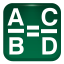# Proportions Calculator Examples

In the examples the green text indicates user entries and the blue text indicates the results. The A, B, C, D indicate the entry positions as in the Proportions Calculator app and as shown on its icon.## Image resize

Resize a 3264x2448 pixel image taken on an 8 megapixel camera to fit on a website where there is room for the image to be 216 pixels tall.

 3264 288 2448 216

A = 3264 (width)
B = 2448 (height)
C = result
D = 216 (desired height)

## Video size reduction

Reduce a 1080i video to standard definition width.

 1920 720 1080 405

A = 1920 (width)
B = 1080 (height)
C = 720 (desired width)
D = result

## Find tip amount

For a \$48.40 dinner find the 15% tip amount.

 15 7.26 100 48.4

A = 15 (tip %)
B = 100 (%)
C = result
D = 48.40 (dinner amount)

## Calculate sales tax amount

Calculate the 7.5% sales tax amount for a \$32.40 purchase.

 7.5 2.43 100 32.4

A = 7.5 (tax %)
B = 100 (%)
C = result
D = 32.40 (purchase amount)

## Scale a recipe

If 2 1/2 cups of pancake mix makes 8 pancakes how much mix is needed for 12 pancakes.

 8 12 2.5 3.75

A = 8 (# pancakes)
B = 2.5 (cups of mix)
C = 12 (needed # pancakes)
D = result

## Convert currency

Convert U.S. \$24 to British Pounds with an exchange rate of 0.66.

 0.66 15.84 1 24

A = .66 (exchange rate)
B = 1
C = result
D = 24 (U.S. dollars)